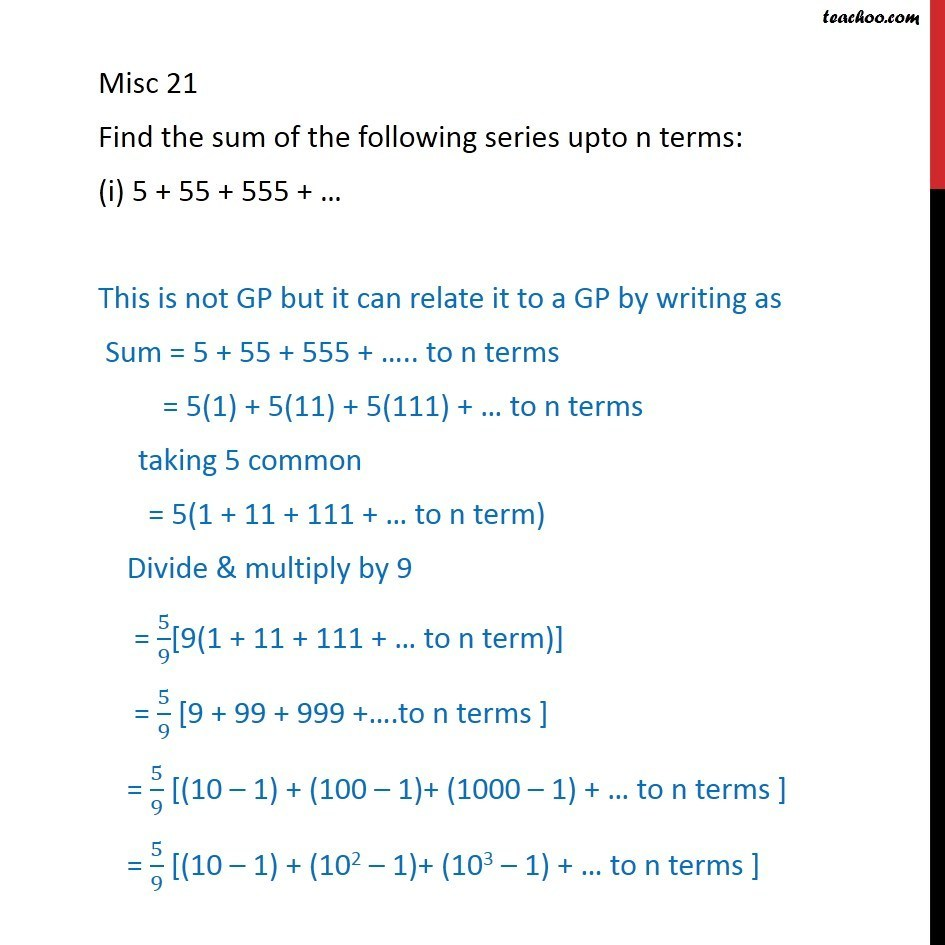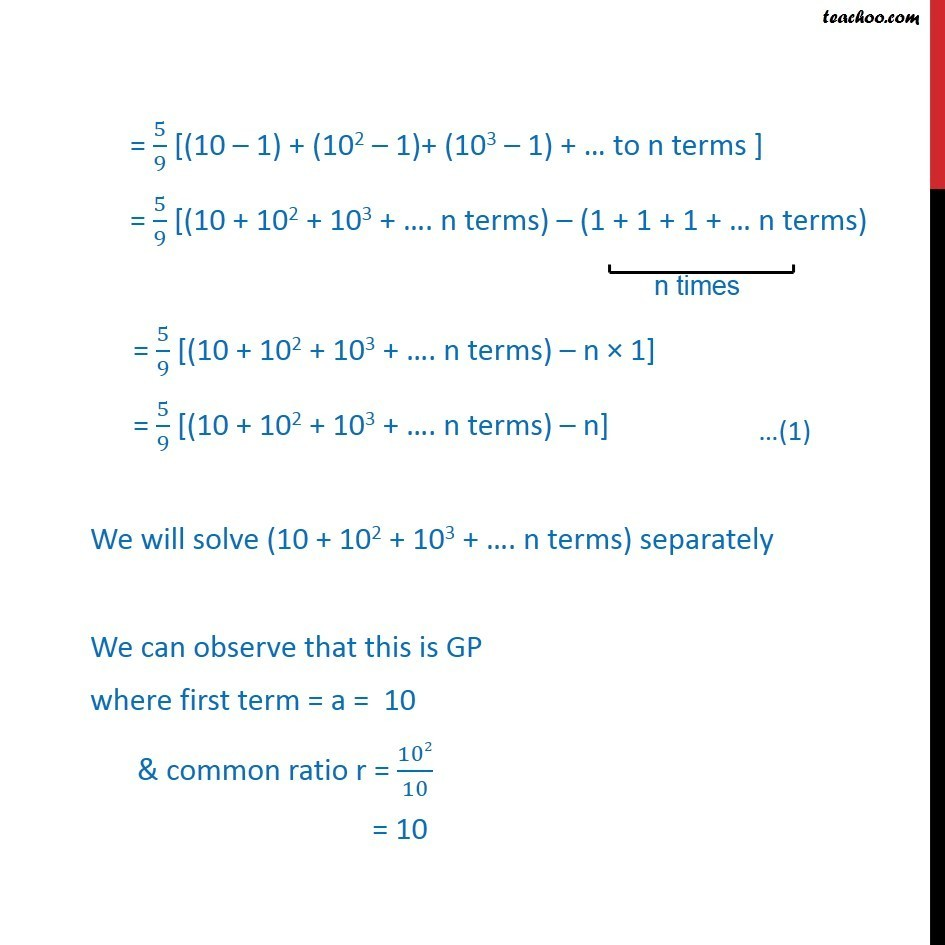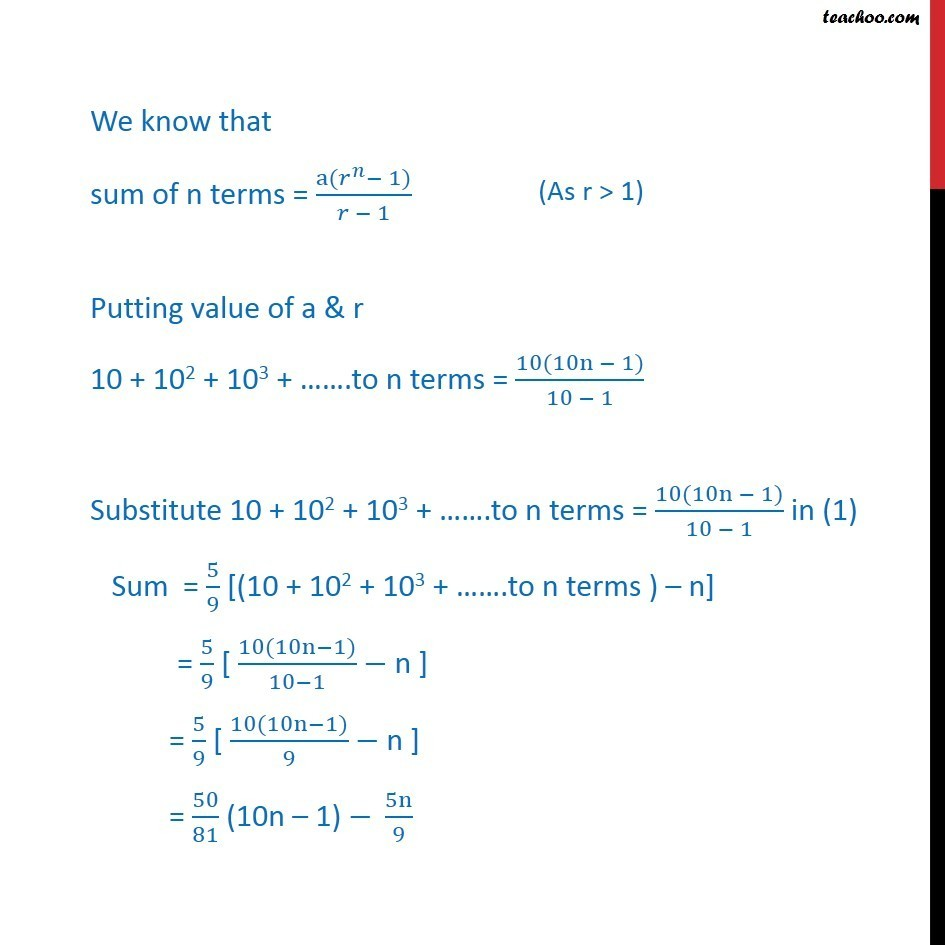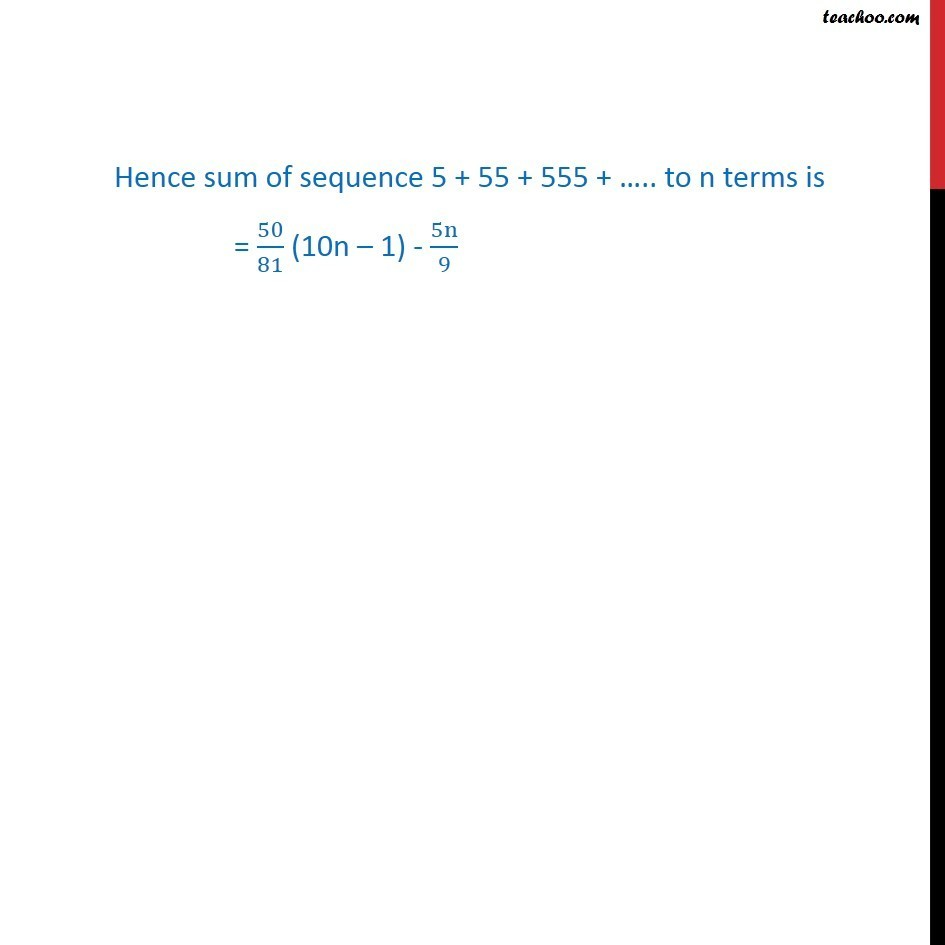Geometric Progression(GP): Formulae based

Chapter 8 Class 11 Sequences and Series
Concept wiseLearn in your speed, with individual attention - Teachoo Maths 1-on-1 Class

### Transcript

Misc 21 Find the sum of the following series upto n terms: (i) 5 + 55 + 555 + … This is not GP but it can relate it to a GP by writing as Sum = 5 + 55 + 555 + ….. to n terms = 5(1) + 5(11) + 5(111) + … to n terms taking 5 common = 5(1 + 11 + 111 + … to n term) Divide & multiply by 9 = 5/9[9(1 + 11 + 111 + … to n term)] = 5/9 [9 + 99 + 999 +….to n terms ] = 5/9 [(10 – 1) + (100 – 1)+ (1000 – 1) + … to n terms ] = 5/9 [(10 – 1) + (102 – 1)+ (103 – 1) + … to n terms ] = 5/9 [(10 – 1) + (102 – 1)+ (103 – 1) + … to n terms ] = 5/9 [(10 + 102 + 103 + …. n terms) – (1 + 1 + 1 + … n terms) = 5/9 [(10 + 102 + 103 + …. n terms) – n × 1] = 5/9 [(10 + 102 + 103 + …. n terms) – n] We will solve (10 + 102 + 103 + …. n terms) separately We can observe that this is GP where first term = a = 10 & common ratio r = 102/10 = 10 We know that sum of n terms = (a(𝑟^𝑛− 1))/(𝑟 − 1) Putting value of a & r 10 + 102 + 103 + …….to n terms = (10(10n − 1))/(10 − 1) Substitute 10 + 102 + 103 + …….to n terms = (10(10n − 1))/(10 − 1) in (1) Sum = 5/9 [(10 + 102 + 103 + …….to n terms ) – n] = 5/9 [ (10(10n−1))/(10−1) − n ] = 5/9 [ (10(10n−1))/9 − n ] = 50/81 (10n – 1) − 5n/9 Hence sum of sequence 5 + 55 + 555 + ….. to n terms is = 50/81 (10n – 1) - 5n/9